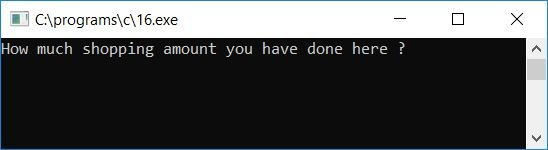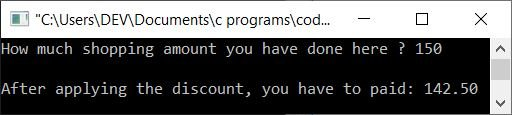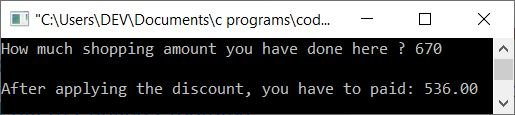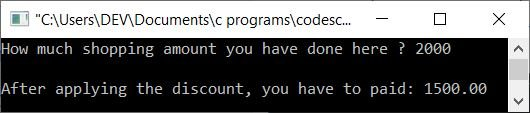# C Program to Determine Purchase Amount After Discount

In this post, we will learn about how to create a program in C that will ask the user to enter the total shopping amount purchased in the shop and then apply the discount as per the following discount criteria, then find and print the final amount that has to be paid by the customer after subtracting the discount amount:

• If the user has purchased something that costs from \$101 to \$200, the discount will be 5%.
• If the user has purchased something that costs from \$201 to \$400, the discount will be 10%.
• If the user has purchased something that costs from \$401 to \$800, the discount will be 20%.
• And if the user has purchased something that costs more than \$800, the total discount that will be applied to the whole purchase amount is 25%.

Let's take a look at the program:

```#include<stdio.h>
#include<conio.h>
int main()
{
float amount, discount, amountToBePaid;
printf("How much shopping amount you have done here ? ");
scanf("%f", &amount);
printf("\n");
if(amount<=100)
printf("You have to paid: %0.2f", amount);
else
{
if(amount>100 && amount<=200)
{
discount = (amount*5)/100;
amountToBePaid = amount-discount;
printf("After applying the discount, you have to paid: %0.2f", amountToBePaid);
}
else if(amount>200 && amount<=400)
{
discount = (amount*10)/100;
amountToBePaid = amount-discount;
printf("After applying the discount, you have to paid: %0.2f", amountToBePaid);
}
else if(amount>400 && amount<=800)
{
discount = (amount*20)/100;
amountToBePaid = amount-discount;
printf("After applying the discount, you have to paid: %0.2f", amountToBePaid);
}
else
{
discount = (amount*25)/100;
amountToBePaid = amount-discount;
printf("After applying the discount, you have to paid: %0.2f", amountToBePaid);
}
}
getch();
return 0;
}```

As the program was written in the Code::Blocks IDE, here is the output you will get on your output screen after a successful build and run. This is the first snapshot of the sample run:Now enter the total amount (the original purchase amount), say \$150. That is, the customer has made a purchase of \$150 at the shop. Now press the ENTER key to see the total cost (final cost) the customer has to pay after applying the discount. Here is the second snapshot of the sample run:Let's take another sample run. This time, the customer made a purchase of \$670. Here is a snapshot of the sample run::Here is another sample run, this time from a customer purchase made in \$2000:Here are some of the main steps used in the above program:

• Receive the original purchase amount.
• Use the if statement to check whether the purchase amount is less than or equal to \$100 or not.
• If it is, then just ask him or her to pay the original purchase amount as the final purchase amount without applying any discount. As the discount starts only when the purchase amount exceeds \$100.
• Otherwise, apply the discount and ask him/her to pay the final, or net, amount after applying the discount if the purchase amount exceeds \$100.
• Here, first, we have to calculate the discount amount. To calculate the discount amount, multiply the amount by the discount percentage, divide it by 100, and then initialize it to a variable that holds the discount value, say, discount. And then minus the discount value from the original purchase amount and initialize it to another variable that holds the final amount that has to be paid by the customer, say amountToBePaid.
• Finally, print the value of amountToBePaid as output. Whatever the net or final amount is, it is stored in the amountToBePaid variable.
• For example, let's suppose the user has purchased a few items that cost \$768. Alternatively, the user has entered 768 as input.
• As the given value is greater than 400 and less than or equal to 800, the discount that will be applied to this purchase amount will be 20%.
• As a result, (amount*20)/100, (768*20)/100, (15360)/100, or 153.6 will be set to discount.
• And amount-discount or 768-153.6 or 614.4 will be initialized to amountToBePaid.
• Now print the value of amountToBePaid as output, which will be the net amount that has to be paid by the customer.

C Quiz

« Previous Program Next Program »